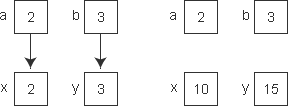# C语言函数的参数和返回值

## 形式参数和实际参数

• 形参变量只有在被调用时才分配内存单元，在调用结束时，即刻释放所分配的内存单元。因此，形参只有在函数内部有效。函数调用结束返回主调函数后则不能再使用该形参变量。
• 实参可以是常量、变量、表达式、函数等，无论实参是何种类型的量，在进行函数调用时，它们都必须具有确定的值，以便把这些值传送给形参。因此应预先用赋值，输入等办法使实参获得确定值。
• 实参和形参在数量上，类型上，顺序上应严格一致，否则会发生类型不匹配”的错误。【例8-2】计算 1+2+3+...+(n-1)+n 的值。

1. #include <stdio.h>
2.
3. int s(int n){
4.     int i;
5.     for(i=n-1;i>=1;i--)
6.         n=n+i;
7.     printf("The inner n = %d\n",n);
8.
9. return n;
10. }
11.
12. int main(void){
13.     int n, total;
14.     printf("Input a number: ");
15.     scanf("%d",&n);
16.     total = s(n);
17.     printf("The outer n = %d \n",n);
18. printf("1+2+3+...+(n-1)+n = %d\n", total);
19.
20. return 0;
21. }

Input a number： 100↙
The inner n = 5050
The outer n = 100
1+2+3+. ..+(n-l)+n = 5050

## 函数的返回值

1) 函数的值只能通过return语句返回主调函数。return语句的一般形式为：
return 表达式;

return (表达式);

2) 函数值的类型和函数定义中函数的类型应保持一致。如果两者不一致，则以函数类型为准，自动进行类型转换。

3) 如函数值为整型，在函数定义时可以省去类型说明。

4) 不返回函数值的函数，可以明确定义为“空类型”，类型说明符为“void”。如【例8-2】中函数s并不向主函数返函数值，因此可定义为：

1. void s(int n){
2. /* …… */
3. }

1. sum=s(n);

(2)
100%

(0)
0%